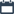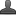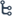Showing posts with label Mathematics trick. Show all posts
Showing posts with label Mathematics trick. Show all posts

## Wednesday, November 11, 2020

•November 11, 2020
••, ,
•2 comments

## Free Vedic maths trick : How to find the answer without knowing the question & person's pocket money

Maths is Interesting !

Hi friends and my dear students! In this post, I have covered  ( A ) How to predict a person's Date of Birth ( B ) How to predict a person's pocket  money( C ) How to find the answer without knowing the question !After Reading  Please do share it with your friends. Learn maths for All classes here

Every second person whom I meet tells me that he hates mathematics . Because they hate mathematics , they get poor scores in their exams and the poor scores further aggravate their hatred . Thus it becomes a vicious circle !

Over the years I realized that people often came to my seminars with a negative mindset towards mathematics . Since an early age , their mind is conditioned to believe that maths is boring and difficult . Thus , it becomes inevitable to create a ' paradigm shift towards the subject . Unless they are excited about the techniques that will follow , it becomes a Herculean task to arouse enthusiasm towards the seminar .

With a desire to create a curiosity and yearning for the subject , we researched on a set of techniques which we call ' Mental Magic . ' These techniques are so exciting and powerful that they leave the audience bewildered . They get tantalized with its workings and their entire attitude towards mathematics changes in a few minutes . After the session on Mental Magic is over , the audience is all eager , excited and ready to grasp the techniques of Vedic Mathematics . This really simplifies my task ....

In this chapter , we are going to study many of these techniques which will change your attitude towards mathematics . A few of these are trade secrets , but for the first time I am revealing them in my In such sessions , I predict the date of birth of anybody from the audience . I also predict strange things like how many brothers / sisters he has , how many children he has , how much money one has in his pockets etc. without him disclosing any information ! I also do a technique where I can get the answer to a problem without even knowing the problem ! Sounds interesting ? ! Read ahead ........

( A ) How to predict a person's Date of Birth .

With this technique you can predict the date of birth of any number of people simultaneously . You can try this stunt with your family members , friends , relatives , colleagues and even in parties .

STEPS

( a ) Ask the people to take the number of the month in which they were born ( January is 1 , February is 2 and so on ..... )

( b ) Next , ask them to double the number

( C ) Add 5 to it

( d ) Multiply it by 5

( e ) Put a zero behind the answer

( f ) Add their date of birth ( If they are born on 5th January then add 5 )

After the steps are over , ask them to tell you the final answer . And lo ! Just by listening to their final answer you can predict their date of birth !

Also Check

## Vedic maths trick :Squaring of numbers ending with ' 5

SECRET

From the answer that you get from each member of the audience ,

• Mentally subtract 50 from the last two digits and you will have the date

• Subtract 2 from the remaining digits and you will have the month .

Thus , you will easily get his date of birth . 17

Example :

Let us suppose I was a member of the audience . My date of birth is 26th June . Then I would have worked out the steps as follows :

( a ) Take the month number    = 6

( b ) Double the answer            = 12

( c) Add 5 to it                          = 17

( d ) Multiply it by 5                 = 85

( e ) Put a zero behind the answer = 850

( f) Add the date of birth = 850 + 26 = 876

Thus my final answer is 876. Now , let us see how we can deduct my date of birth from the final answer . We will subtract 50 from the last two digits to get the date . Next , we will subtract 2 from the remaining digits to get the month .

8   |    76

----------------------

- 2   |    50

----------------------

6   |     26

---------------------

( month )    ( date )

On similar lines if the total was 765 , 1481 and  the birthdates would be 15th May , 31th December and 21st August respectively .

7   |      65

---------------------

-2  |     50

---------------------

5  |    15

--------------------

( month )    ( date )

14         |       81

-----------------------

-2         |     50

----------------------

12           |     31

----------------------

( month )  -  ( date )

With the knowledge of this technique , you can predict the birth - date of hundreds of people simultaneously .

( Note : There are many such mathematical ways by which you can predict a person's date of birth but the method given above is one of the simplest methods )

( B ) How to predict a person's pocket money

This technique will help you to find how much money a person has in his pocket / wallet etc. It can be tried on a group of people simultaneously .

STEPS

( a ) Ask him to take the amount he has in his pocket ( just the rupees , ignore the paisa )

( b ) Next , ask him to add 5 to it .

( C ) Multiply the answer by 5

( d ) Double the answer so obtained

( e ) Finally , ask him to add his favourite one digit number ( from 0-9 )

After the steps are over ask them to tell you the final answer . And just by listening to the final answer you will come to know the amount he has in his pocket !

SECRET

• Ignore the digit in the unit's place .

• From the remaining number , subtract 5 and you will come to know the amount he has in his pocket .

Example :

Let us suppose a person has 20 Rupees in his pocket . He would work out the steps as given below :

( a ) Tike the amount in your pocket                             = 20

( b ) Add 5                                                                       = 25

( c ) Multiply by 5                                                           = 125

( d ) Double the answer                                                 =250

( e ) Add your favourite single digit number ( say , 7 ) =257

Thus , his final answer would be 257. Now , let us see how we can find out the amount he has from the final answer . As mentioned earlier , we will ignore the digit in the unit's place in this case it is 7 ) . Now the remaining number is 25. From 25 , we subtract 5 and get the amount as Rs . 20. And thus our answer is confirmed .

Similarly if the total was 1052 , 53 and 160 the amount would be 100 , 0 and 11 Rupees respectively .

( From 1052 , we ignore the last digit 2 and take only 105. From 105 , we subtract 5 and get the answer as 100 and so on ... )

I use this technique in my seminars to give the audience some relief from complex calculations . There are many ways by which we can predict the amount a person has in his pocket but I prefer this technique because it neither involves any complex calculations nor is it too obvious for the audience to guess the secret .

( C ) How to find the answer without knowing the question !

Using this technique you can find the total of a set of five numbers without knowing the numbers . In my seminars , I ask a member of the audience to give me a three - digit number . Let us suppose someone he gives me the number as 801. Then , I write the number 801 and after leaving four lines I write the final answer

801 ( Audience )

Ans : Thus , I have got the final answer without knowing all the numbers . Next , I ask him to give me another three digit number . Let us suppose he gives me 354. Now , its my turn . Within one second , I write th

801 ( Audience )

354 ( Audience )

645 ( Speaker )

Ans : 2799

Now , again it's the turn of the audience . Let us suppose he gives me 800. Now , again it is my turn . Before he blinks his eye , I write my number as 199 .

801 ( Audience )

354 ( Audience )

645 ( Speaker )

800 ( Audience )

199 ( Speaker )

Ans : 2799

Thus , I have all five numbers in place . When I ask him to check the total of the five numbers , he is amazed to find out that it is 2799 which I had predicted by looking at the first number only !

Thus , I got the answer without knowing the question . Further , after every step I was writing my three - digit number within one second ( without any time for calculation )

Even you can do such ' Magic Totals . You can ask the audience to give you any three - digit number and based on that you can get the final total without looking at the other numbers .

SECRET .

From the first number that the audience gives you , subtract 2 and always put 2 in the beginning . This becomes your final answer . For example , if the number is 801 , we subtract 2 and get 799. Next , we put 2 in the beginning and our final answer becomes 2799. If the number is 567 , we subtract 2 and get 565. Next , we put 2 in the beginning and write the final answer as 2565 . Thus , you will have the final answer .

• Next , we will see the secret of finding the subsequent steps . Subtract each digit of the number that the audience gives you from 9 and you will have your number . In the example mentioned above , when the audience gives me 354 , I subtract each of the digits 3 , 5 and 4 from 9 and get my answer as 6 , 4 and 5. So my number is 645. When the audience gives me the number 800 , I subtract each of the digits 8,0 and 0 from 9 and get my answer as 1 , 9 and 9. So my number is 199 .

In this way , you can have the intermediary steps .

Let us have a look at another example .

600 ( A )          600 ( A )            600 ( A )

-----  481 ( A )    --     481  ( A )

518 ( S )            518 ( S )

009 ( A )

090 ( S )

Ans : 2598       Ans : 2598

( A = Audience , S = Speaker )

If the audiet gives you a mber as 600 , you will tract 2 from it ( 598 ) and put 2 in the beginning . Thus , your final answer is 2598. Next , let us assume the audience gives you the number 481. We subtract each of the digits 4 , 8 and 1 from 9 and get our answer as 518 instantly . Next , let us suppose the audience gives you the number 909. We subtract each of the digits 9,0 and 9 from 9 and Ans : 2598

get our answer as 090. When you check the final total , you will be surprised to find that it is indeed 2598 !

Thus , I have taught you three exciting techniques of mathematics which you can use to impress your friends and colleagues . The intention of including this chapter in the book was twofold . First , since most people have got habituated with the calculator , it is a good opportunity to tune your mind to mental calculation . Secondly , the wonderful response that you will get by using these techniques on people will definitely change your attitude towards mathematics .

Note : Since the techniques of this chapter fall beyond the scope of Vedic Mathematics , I have not elaborated on them . However , if you are keen on learning many such ‘ mental magic ' techniques like predicting how much many brothers & sisters a person has , what is a person's year of birth and such other techniques , then fill the feedback form given at the end of the book and mail it to me with the words ' Mental Magic ' written on the envelop . I will send across the techniques